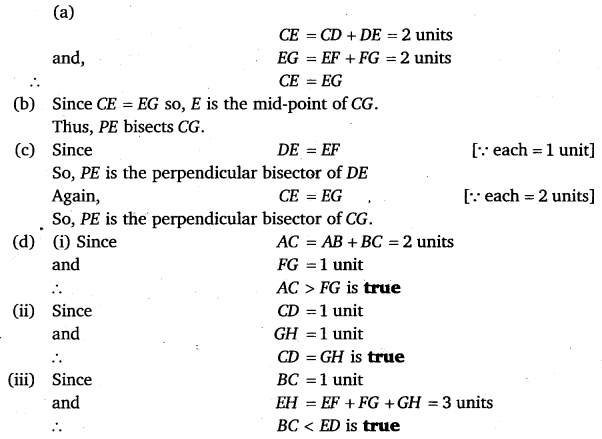# Class 6 Maths NCERT Solutions for Chapter 5 Understanding Elementary Shapes Ex – 5.5

## Understanding Elementary Shapes

Question 1.
Which of the following are models for perpendicular lines:

(a) The adjacent edges of a table top.
(b) The lines of a railway track.
(c) The line segments forming the letter ‘L’.
(d) The letter V.

Solution:
(a) Yes
(b) No
(c) Yes
(d) No

Question 2.
Let$\overline { PQ }$ be the perpendicular to the line segment$\overline { XY }$. Let$\overline { PQ }$ and$\overline { XY }$ intersect in the point A. What is the measure of ∠PAY?

Solution:
The measure of ∠PAY =90° [∵ PQ ⊥ XY, given]Question 3.
There are two “set-squares” in your box. What are the measures of the angles that are formed at their corners? Do they have any angle measure that is common?

Solution:
In one, the angles are of 30°, 60°, 90° and in the other the angles are of 90°, 45°, 45°.
Clearly, the common angle = 90°

Question 4.
Study the diagram. The line l is perpendicular to line m.

(a) Is CE = EG?
(b) Does PE bisect CG?
(c) Identify any two line segments for which PE is the perpendicular bisector. ‘ ’
(d) Are these true?
(i) AC > FG
(ii) CD = GH
(iii) BC< EH

Solution: